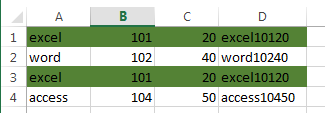# How to Find and Highlight Duplicate Rows in Excel

This post will guide you how to find and highlight duplicate rows in a range of cells in Excel. How do I find duplicate rows in a range with a formula in Excel. How to find duplicate rows in two or three columns with Conditional Formatting feature in Excel.

## Find Duplicate Rows Only

If you have a list of data in two or more columns, such as: A1:C4, and you want to find duplicate rows in those columns in Excel. How to achieve it. You can use a formula based on the IF function, the SUMPRODUCT function to achieve the result of finding all duplicate rows in a range of cells. If found, returns TRUE, otherwise, returns False. Like this:

`=IF(SUMPRODUCT((\$A\$1:\$A\$4=A1)*1,(\$B\$1:\$B\$4=B1)*1,(\$C\$1:\$C\$4=C1)*1)>1,"TRUE","FALSE")`

Type this formula into Cell D1, and press Enter key in your keyboard, and then drag the AutoFill Handle over to other cells to apply this formula.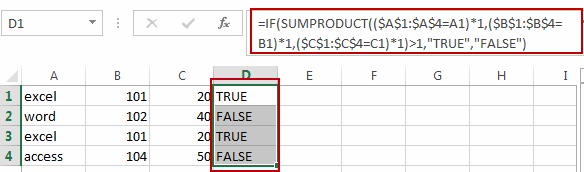## Find and Highlight Duplicate Rows

If you want to highlight duplicate rows, you need to use the conditional formatting feature to achieve the result in Excel. You just need to do the following steps:

#1 you need to create a helper column and concatenate values from all columns. Just add the below formula in Cell D1, and press Enter key, then drag the AutoFill Handle over to Cell D4 to apply the formula:

`=A1&B1&C1`

Or

`=CONCATENATE(A1,B1,C1)`

If you have a large number of columns, you can use the TEXTJOIN function to concatenate values for all columns. Like this:

`=TEXTJOIN(“,”,TRUE,A1:X1)`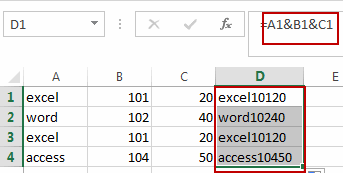#2 select the range of Cells that you want to highlight duplicate rows including the range D1:D4 in Helper column, and go to HOME tab, click Conditional Formatting command under Styles group. And click New Rule from the drop down menu list. And the New Formatting Rule dialog will open.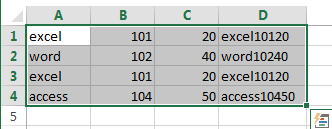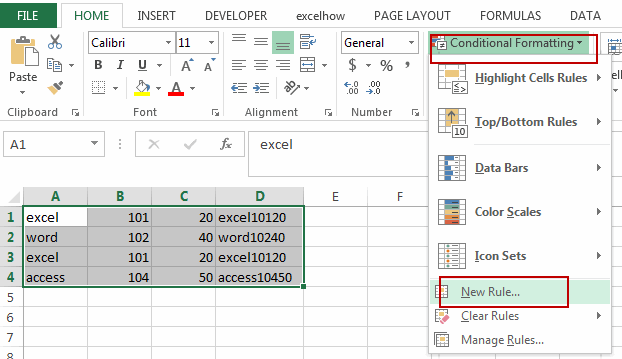#3 select Use a formula to determine which cells to format in Select a Rule Type list box, and type the following formula in text box of the Format values where this formula is true.

`=COUNTIF(\$D\$1:\$D\$4,\$D1)>1`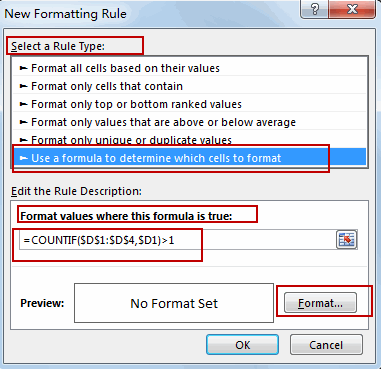D1:D4 is a range of cells in helper column.

#4 click Format command, and the Format Cells dialog will open. And switch to Fill tab, select one background color as you need, then click OK button.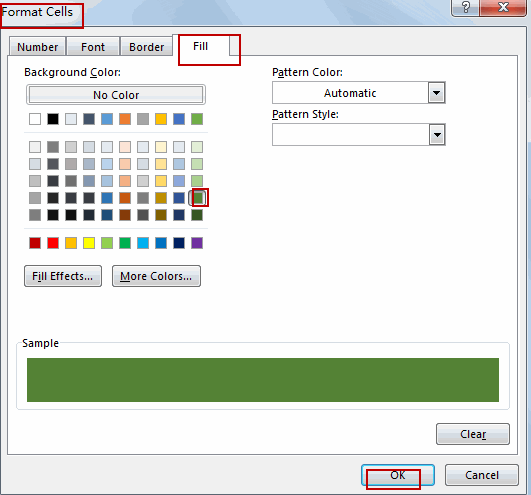#5 click OK button. All duplicate rows are highlighted successfully.Video: Find and Highlight Duplicate Rows

### Related Functions

• Excel SUMPRODUCT function
The Excel SUMPRODUCT function multiplies corresponding components in the given one or more arrays or ranges, and returns the sum of those products.The syntax of the SUMPRODUCT function is as below:= SUMPRODUCT (array1,[array2],…)…
• Excel IF function
The Excel IF function perform a logical test to return one value if the condition is TRUE and return another value if the condition is FALSE. The IF function is a build-in function in Microsoft Excel and it is categorized as a Logical Function.The syntax of the IF function is as below:= IF (condition, [true_value], [false_value])….
• Excel Concat function
The excel CONCAT function combines 2 or more strings or ranges together.This is a new function in Excel 2016 and it replaces the CONCATENATE function.The syntax of the CONCAT function is as below:=CONCAT (text1,[text2],…)…
• Excel TEXTJOIN function
The Excel TEXTJOIN function joins two or more text strings together and separated by a delimiter. you can select an entire range of cell references to be combined in excel 2016.The syntax of the TEXTJOIN function is as below:= TEXTJOIN  (delimiter, ignore_empty,text1,[text2])…
• Excel COUNTIF function
The Excel COUNTIF function will count the number of cells in a range that meet a given criteria. This function can be used to count the different kinds of cells with number, date, text values, blank, non-blanks, or containing specific characters.etc.= COUNTIF (range, criteria)…